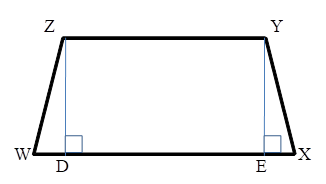Chapter 4.4, Problem 20EElementary Geometry For College St...

7th Edition
Alexander + 2 others
ISBN: 9781337614085

Solutions

Chapter
SectionElementary Geometry For College St...

7th Edition
Alexander + 2 others
ISBN: 9781337614085
Textbook Problem

In trapezoid WXYZ with bases Z Y ¯ and W X ¯ , ZY = 12, YX = 10, WZ = 17, and ZD = 8. Find the length of base W X ¯ .Exercises 19, 20

To determine

To Find:

The length of the base of a trapezoid.

Explanation

Consider the following trapezoid.

Given: ZY = 12, YX = 10, WZ = 17 and ZD = 8.

Consider the ΔDWZ,

According to Pythagoras theorem, (WZ)2=(WD)2+(ZD)2.

Now, (WD)2=(17)2(8)2=28964=225.

Then, WD = 15.

Now consider the ΔEXY,

Here, ZD = YE = 8 and ZY = DE = 12

Still sussing out bartleby?

Check out a sample textbook solution.

See a sample solution

The Solution to Your Study Problems

Bartleby provides explanations to thousands of textbook problems written by our experts, many with advanced degrees!

Get Started

In problems 1-16, solve each equation. 7. Solve

Mathematical Applications for the Management, Life, and Social Sciences

In Exercises 4562, find the values of x that satisfy the inequality (inequalities). 47. 4x 20

Applied Calculus for the Managerial, Life, and Social Sciences: A Brief Approach

Differentiate. f() = cos sin

Single Variable Calculus: Early Transcendentals

Sometimes, Always, or Never: limxaf(x)g(x)=limxaf(x)limxag(x).

Study Guide for Stewart's Single Variable Calculus: Early Transcendentals, 8th

True or False: is a convergent series.

Study Guide for Stewart's Multivariable Calculus, 8th